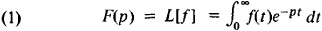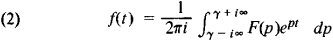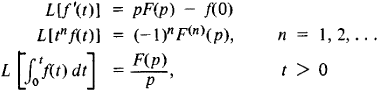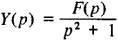# Laplace Transform

(redirected from S transform)

## Laplace transform

[lə′pläs ′trans‚fȯrm]
(mathematics)
For a function ƒ(x) its Laplace transform is the function F (y) defined as the integral over x from 0 to ∞ of the function e -yxƒ(x).

## Laplace Transform

a transformation that converts the function f(t) of a real variable t (0 < t < ∞), called the original, into the functionof the complex variable ρ = σ + i τ. The term “Laplace transform” refers not only to the transform but also to the transformed function F(p). The integral on the right-hand side of (1) is called the Laplace integral. It was examined by P. Laplace in a number of studies brought together in the book Théorie analytique des probabilités, published in 1812. These integrals were used much earlier (1737) by L. Euler in the solution of differential equations.

Under certain conditions (see text below) the Laplace transform determines the function f(t) uniquely. In the simplest cases, f is given by the inversion formulaThe Laplace transform is a linear integral operator. Some of the fundamental formulas that involve the Laplace transform areThe Laplace transform is used in conjunction with the inversion formula (2) in the integration of differential equations. In particular, the definition (1) of the Laplace transform and its linearity imply that the Laplace transform of the solution of an ordinary linear differential equation with constant coefficients satisfies an algebraic equation of the first degree and can therefore be easily found. For example, if y″ + y = f(t), y(0) = y′ (0) = 0, and Y(p) = L[y] and F(p) = L[f], then

L[y″] = p2Y(p)

and

p2Y(p) + Y(p) = F(p)

so thatMany problems in electrical engineering, hydrodynamics, mechanics, and heat conduction are effectively solved using the Laplace transform.

The Laplace transform has been particularly widely used to provide a theoretical basis for the operational calculus, in which the Laplace transform F(p) of f(t) is usually replaced by the function pF(p).

The modern general theory of the Laplace transform is based on the Lebesgue integral. In order that the Laplace transform be applicable to the function f(t), it is necessary that f(t) be Le-besgue-integrable on any finite interval (0, t), for t > 0, and that the integral (1) for f converge at at least one point p0 = σ0+ 0. If the integral (1) converges at the point p0, then it converges at every point p for which Re (pp0) > 0. Thus, if the integral (1) converges at at least one point p0 of the plane, it either converges in the entire plane or there exists a number σc such that the integral converges when Re p > σc and diverges when Re p < σc. The number σc is called the abscissa of convergence of the Laplace integral. F(p) is an analytic function in the half-plane Re p > σc.

### REFERENCES

Ditkin, V. A., and P. I. Kuznetsov. Spravochnik po operatsionnomu ischisleniiu. Osnovy teorii i tablitsy formul. Moscow-Leningrad, 1951.
Kitkin, V. A., and A. P. Prudnikov. Integral’nye preobrazovaniia i operatsionnoe ischislenie. Moscow, 1961.
Doetsch, G. Rukovodstvo k prakticheskomu primeneniiu preobrazovaniia Laplasa. Moscow, 1965. (Translated from German.)
Site: Follow: Share:
Open / Close